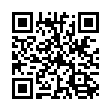# North Coast Synthesis Ltd.

## F♭♭5+2+4Choose audio sample set:
MSK 007 Leapfrog VCF (patch; see in online store)
MSK 009 Coiler VCF (patch; see in online store)

Equivalent chord symbols: E4+2+♯4, E4+9+♯4, E4+2+♯11, F♭♭5+4+9, G♭9-5+♯2, F♭♭5+2+11.

Notes in this chord: F♭, G♭, A♭, B♭♭, C♭♭. Integer notation: {4, 6, 8, 9, 10}.

Nearby chords (one less note): E4+2, F♭♭5+2, F♭♭5+4, A♭2+7+♯1, Esus4♭5+2.

Nearby chords (one more note): E11♭5, EM11♭5, G♭9+♯2, A♭9♯5+♯1, E+2+4+♯4, Edim+2+4+♯3, F♭♭5+2+4+♯1.

Parallel chords (same structure, different root): C♭♭5+2+4, D♭♭5+2+4, E♭♭5+2+4, G♭♭5+2+4, A♭♭5+2+4, B♭♭5+2+4, C♯♭5+2+4, D♯♭5+2+4, E♯♭5+2+4, F♯♭5+2+4, G♯♭5+2+4, A♯♭5+2+4, B♯♭5+2+4.

Experimental fretting charts for guitar standard EADGBE tuning (change tuning or instrument):